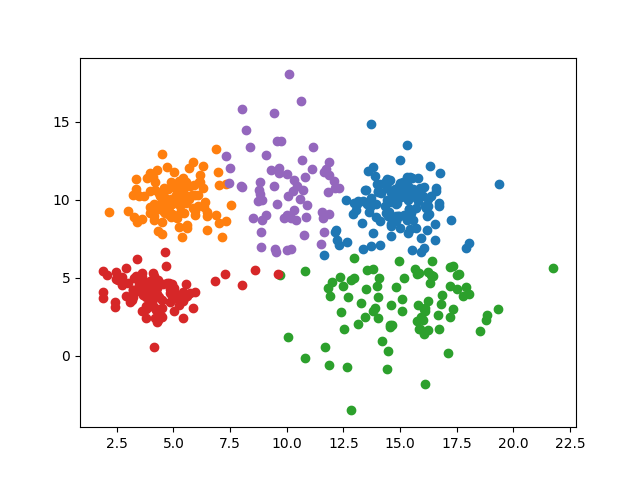# Clustering algorithmsClustering is a form of unsupervised learning, where labels are inferred from similarities in the data.

## K means

K-means is an iterative clustering algorithm that classifies unlabeled into K different classes by finding K mean values best representing the given dataset. Let $K$ be the number of clusters $i ∈ {1, 2, 3, . . . K}, i$ represent each individual cluster and the mean value assigned to it, and $D$ represent the dataset to perform the K-means clustering on:

1. Initiate the algorithm by selecting $K$ random values from the dataset, this will be the initial values $k_i$ for each cluster $i$.
2. Assign each value $v ∈ D$ from the dataset to cluster $i$ where the distance $|v − k_i|$ is minimized.
3. Calculate the cluster mean $k_i$ as the mean value of the data assigned to each cluster.
4. repeat step 2, 3 until a condition is met, commonly this can be that no value $v ∈ D$ changes it‘s associated cluster, a certain number of iterations, or that a cost function has been minimized.
5. Post convergence replace the value of each point to the value of it‘s associated cluster

## Spectral clustering

The spectral clustering algorithm uses a similarity measure (similarity matrix $S$) such as k-nn, or Gaussian similarity. By building the laplacian matrix defined as: $$L = S - D$$ Where $L$ is the graph laplacian, $S$ is the similarity matrix of choice, and $D$ is a diagonal matrix where each diagonal $D_{ii}$ corresponds to the sum $\sum_{j=0}^n S_{ij}$. zero eigenvalues corresponds to the number of connected components (clusters disconected from each other will give several zero eigenvalues). So eigenvalues close to zero then indicates that some components has a weak conection to another component. Each column $j$ of the laplacian matrix is the eigenvector corresponding to the $j$-th eigenvalue of the laplacian. By sampling the $K$ eigenvectors with close to zero eigenvalues it will create a domain with higher seperability between the $K$ clusters. The labels can be extracted by performing K-means clustering in the new domain.